# Basic features of indefinite integral

These features are used to make integral transformation to the elementary one which can easily be integrated.

## 1. Derivative of indefinite integral equals to subintegral function: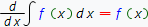## 2. Differential of indefinite integral equals to subintegral expression: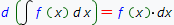## 3. Indefinite integral of differential of some function equals to the sum of this function and arbitrary constant:## 4. Constant multiplier can be factored out of integral: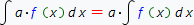, so a ≠ 0

## 5. Integral of sum (difference) equals to the sum (difference) of integrals: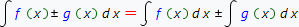## 6. Feature is the linear combination of features 4 and 5: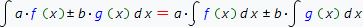, so a ≠ 0 ˄ b ≠ 0

## 7. Invariance feature of indefinite integral:

If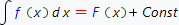, then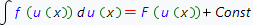## 8. Feature:

If, then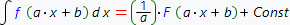If fact, this feature is the particular case of integration by substitution method , which described in details in next chapter.

## Example: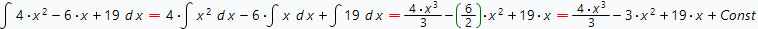At first we apply feature 5, then feature 4, then we use primitives table and get the result.

Our online integrals calculator supports all the features described above and will find the step by step solution for your integral.